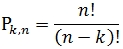# SQL Server LPERMUT function

LPERMUT
Updated: 30 May 2017

Use the SQL Server scalar function LPERMUT to calculate the natural logarithm of the PERMUT function. The PERMUT function calculates the number of permutations without replacement defined as:Syntax
SELECT [wct].[LPERMUT] (
<@n, int,>
,<@k, int,>)
Arguments
 Input Name Definition @n Number of items @k Number chosen
Return Type
float
Remarks
• If @n < 0 then NULL is returned.
• If @k < 0 then NULL is returned.
• If @k > n then NULL is returned.
• Available in XLeratorDB / statistics 2008 only
Examples
Example #1
SELECT wct.LPERMUT(10,3) as LPERMUT

This produces the following result.

Example #2

Calculate the probability of 2 people having the same birthday in a room containing n people.

SELECT
x.n
,1 - EXP(wct.LPERMUT(365,x.n)-wct.LPERMUTA(365,x.n)) as [p(n)]
FROM (VALUES
(10),(20),(22),(23),(30),(57))x(n)

This produces the following result.Copyright 2008-2021 Westclintech LLC         Privacy Policy        Terms of Service